Examples

Chapter 4 Class 11 Mathematical Induction
Serial order wise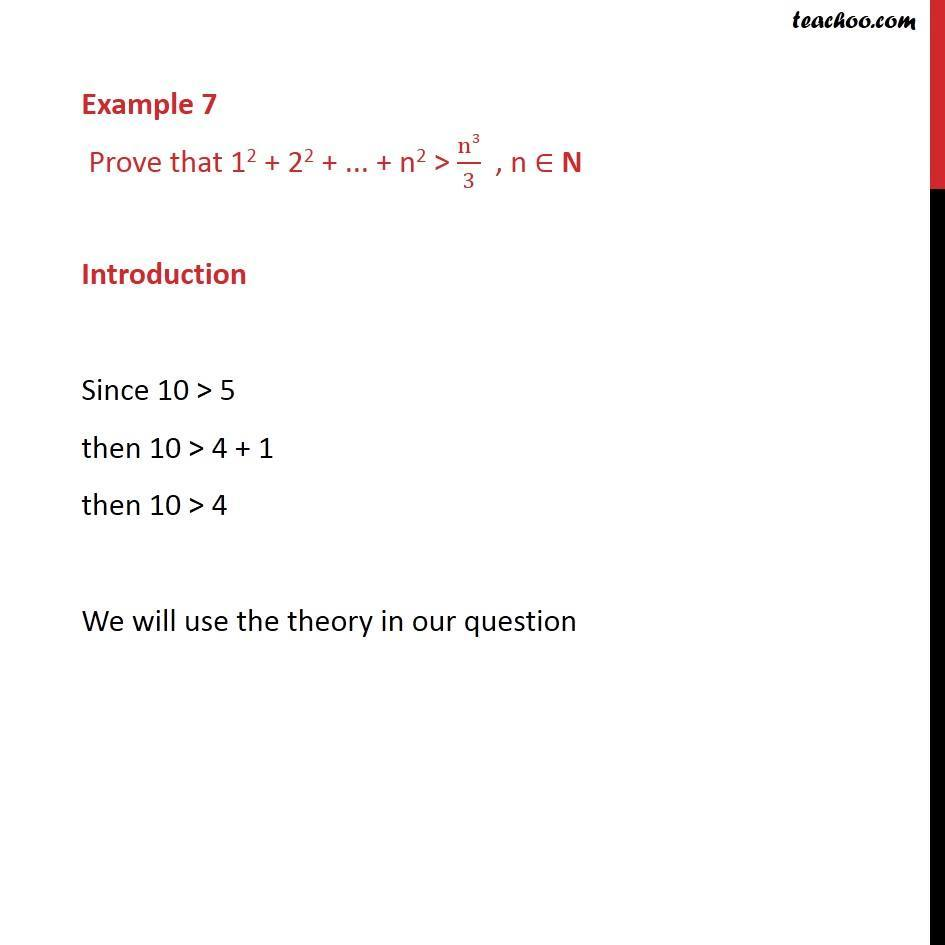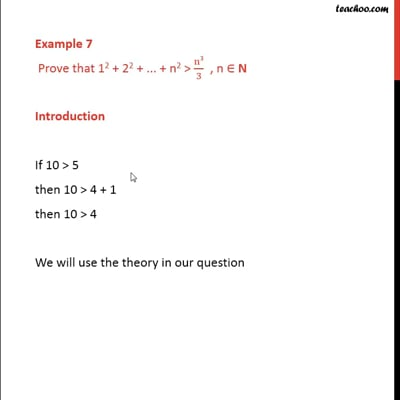This video is only available for Teachoo black users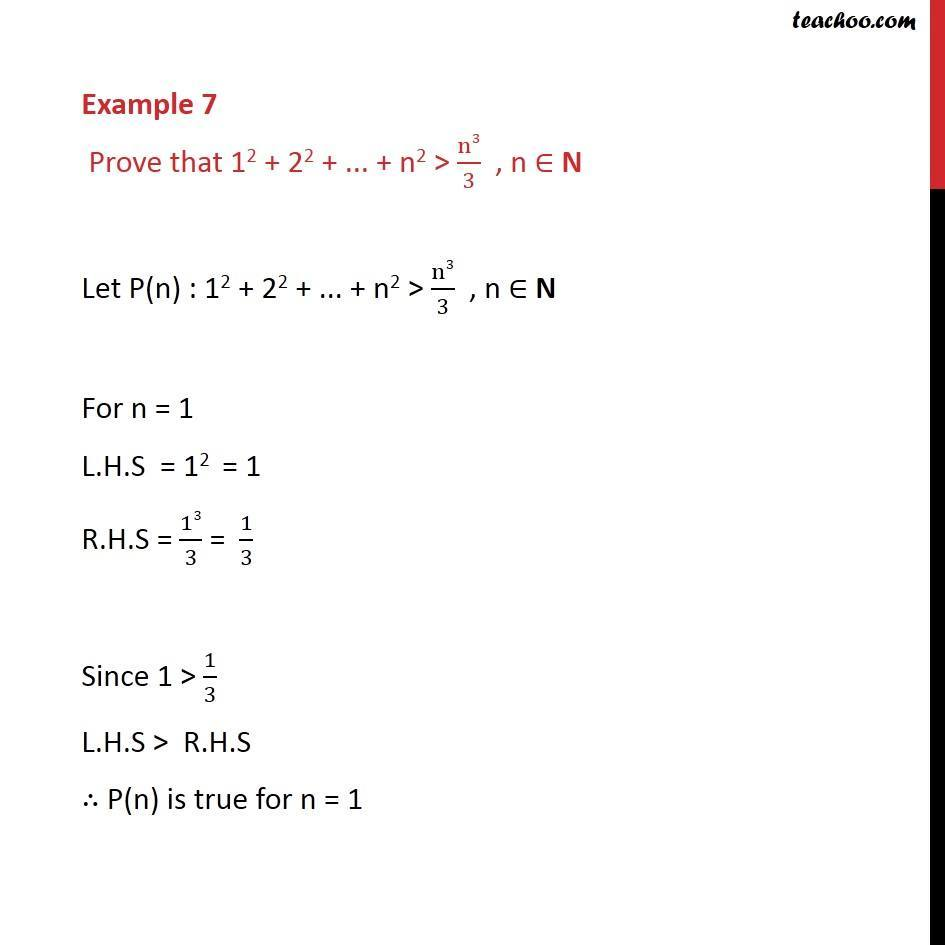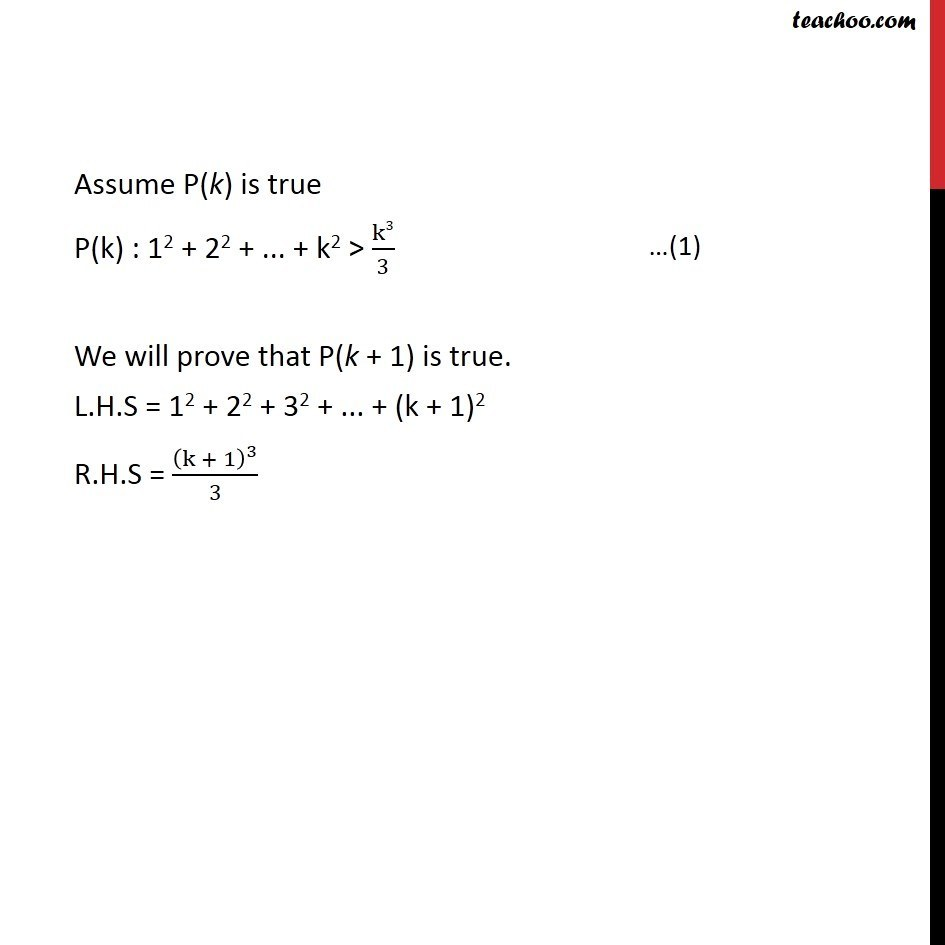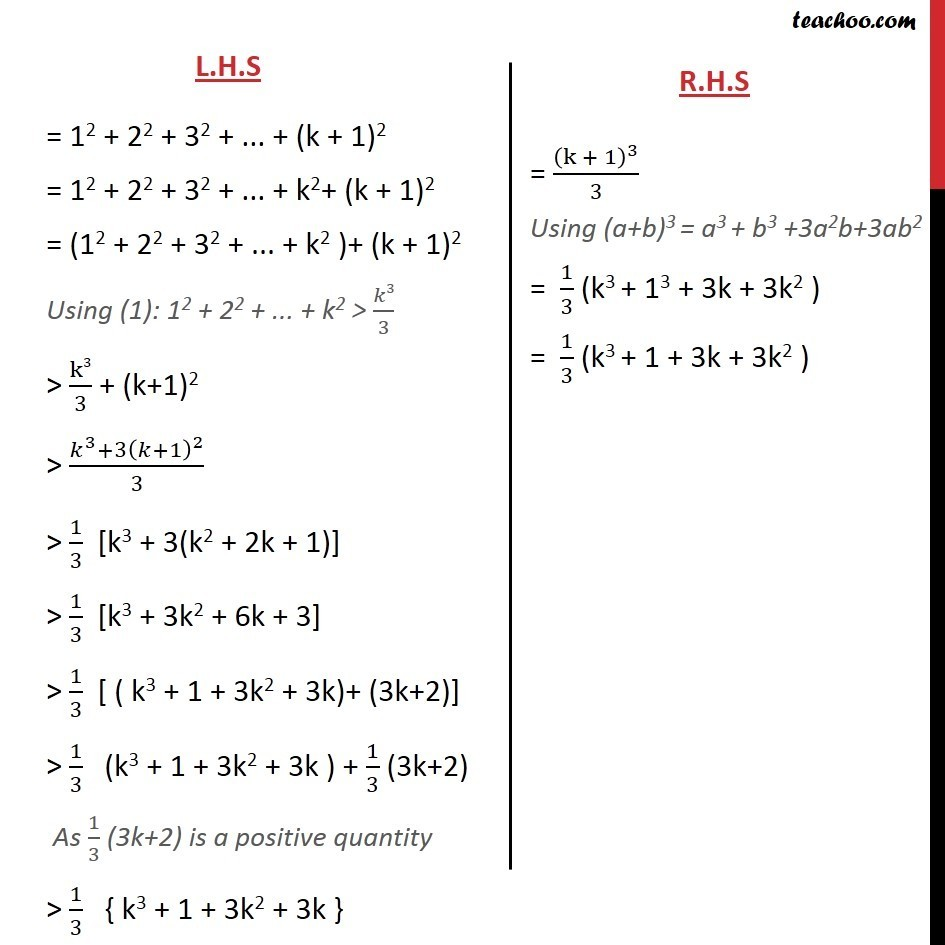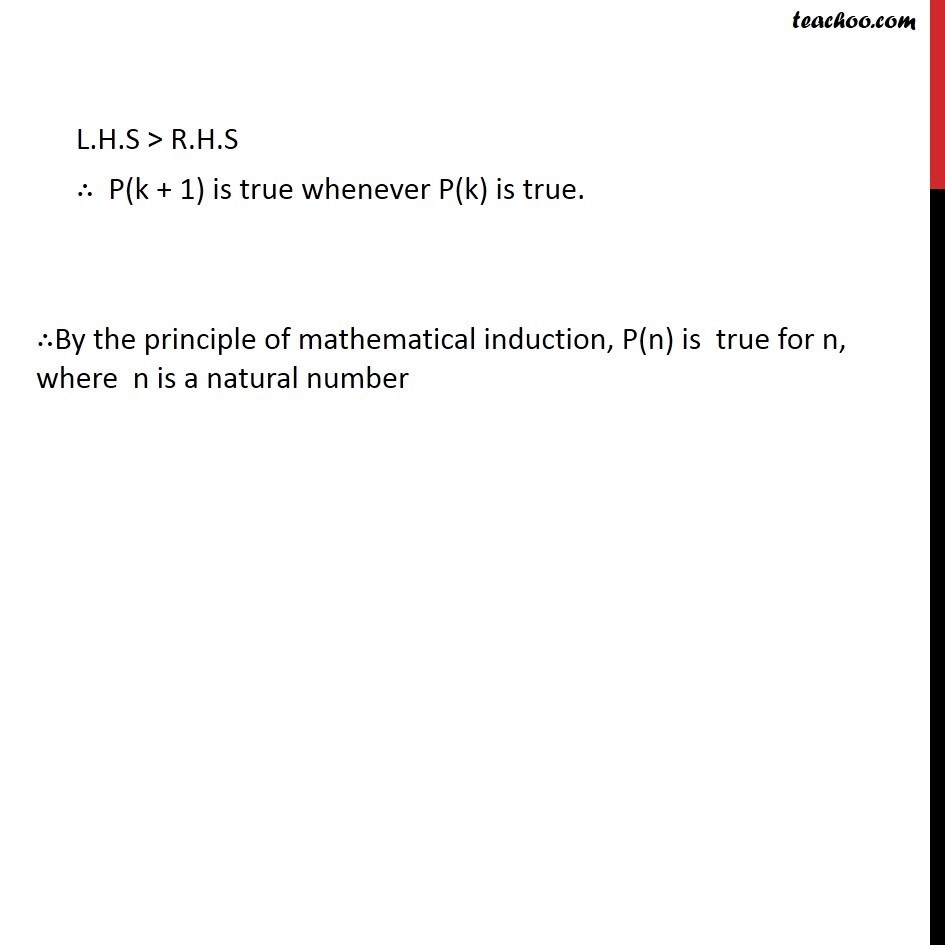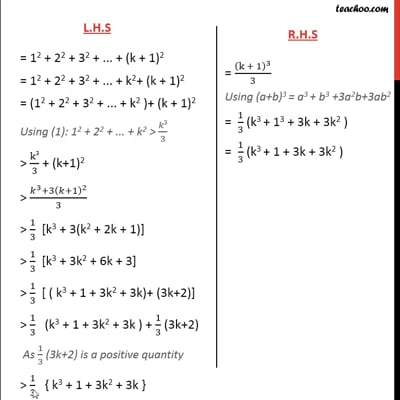This video is only available for Teachoo black users

Solve all your doubts with Teachoo Black (new monthly pack available now!)

### Transcript

Example 7 Prove that 12 + 22 + ... + n2 > n3/3 , n ∈ N Introduction Since 10 > 5 then 10 > 4 + 1 then 10 > 4 We will use the theory in our question Example 7 Prove that 12 + 22 + ... + n2 > n3/3 , n ∈ N Let P(n) : 12 + 22 + ... + n2 > n3/3 , n ∈ N For n = 1 L.H.S = 12 = 1 R.H.S = 13/3 = 1/3 Since 1 > 1/3 L.H.S > R.H.S ∴ P(n) is true for n = 1 Assume P(k) is true P(k) : 12 + 22 + ... + k2 > k3/3 We will prove that P(k + 1) is true. L.H.S = 12 + 22 + 32 + ... + (k + 1)2 R.H.S = (k + 1)^3/3 L.H.S = 12 + 22 + 32 + ... + (k + 1)2 = 12 + 22 + 32 + ... + k2+ (k + 1)2 = (12 + 22 + 32 + ... + k2 )+ (k + 1)2 Using (1): 12 + 22 + ... + k2 > 𝑘3/3 > k3/3 + (k+1)2 > (𝑘^3+3(𝑘+1)^2)/3 > 1/3 [k3 + 3(k2 + 2k + 1)] > 1/3 [k3 + 3k2 + 6k + 3] > 1/3 [ ( k3 + 1 + 3k2 + 3k)+ (3k+2)] > 1/3 (k3 + 1 + 3k2 + 3k ) + 1/3 (3k+2) As 1/3 (3k+2) is a positive quantity > 1/3 { k3 + 1 + 3k2 + 3k } R.H.S = (k + 1)^3/3 Using (a+b)3 = a3 + b3 +3a2b+3ab2 = 1/3 (k3 + 13 + 3k + 3k2 ) = 1/3 (k3 + 1 + 3k + 3k2 ) L.H.S > R.H.S ∴ P(k + 1) is true whenever P(k) is true. ∴By the principle of mathematical induction, P(n) is true for n, where n is a natural number